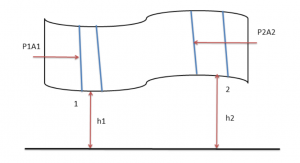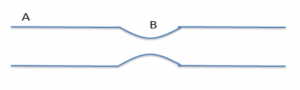# FAQ on Bernoullis Principle – Conservation of Energy

Below we discussed have some of the most frequently asked questions about Bernoulli’s principle and students will also find answers to these questions.

## Important Questions on Bernoulli’s Principle

1. What is the Bernoulli’s Principle?

Ans: Bernoulli’s principle is based on the principle of conservation of energy. According to Bernoulli’s principle, In fluid dynamics, for a non – viscous, incompressible, non – conducting fluid, an increase in the velocity of the fluid during its flow (laminar), results in a simultaneous decrease in pressure (or potential energy) of the fluid. The converse of this is also true.

2. What is the Bernoulli’s Equation?

Ans: Bernoulli’s equation is based on Bernoulli’s Principle for fluid flow. According to Bernoulli’s equation for a fluid, we can see that the total energy of the system is always constant. It is expressed as per the relation which is given below.

$P\;+\;\frac{1}{2} \rho v^2\;+\;\rho gh\;=\;constant \;\;for\; \;a\;\; system$

where,

$P$ is the Pressure Energy

$\frac{1}{2} \rho v^2$ is the Kinetic Energy of the system per unit volume

$\rho gh$ is the Potential Energy per unit volume

Suppose there is an irrotational and steady flow of an incompressible and non-viscous liquid as shown in the figure below. There is a tube of varying cross section and varying height. Consider a liquid contained between the cross sections 1 and 2 of the tube. The heights of 1 and 2 are h1 and h2 respectively from a reference level. This liquid advances into the tube and after a time t is contained between the cross sections 1 and 2.$P_1 +\frac{1}{2}pv_1^2+ \rho gh_1 = P_2 +\frac{1}{2}\rho v_2^2 + \rho gh_2$

Or

$P\;+\;\frac{1}{2} \rho v^2\;+\;\rho gh\;=\;constant\;\; for\;\; a\;\; system$

3. Water flows in a horizontal tube as shown in the figure below. The pressure of water changes by 600 N/m2 between A and B where the areas of cross section are 30 cm2 and 15 cm2 respectively. Find the rate of flow of water through the tube.Ans: Let the velocity at $A$ = $v_A$ and the velocity at $B$ = $v_B$

From the equation of continuity

$\frac{v_B}{v_A} =\frac{30cm^2}{15cm^2}=2$ …………(1)

Applying Bernoulli equation,

$P_A+\frac{1}{2} v_A^2= P_B+\frac{1}{2} \rho v_B^2$

$P_A-P_B=\frac{1}{2}\rho (v_B^2-v_A^2)$

Using equation (1)

$P_A-P_B\;=\;\frac{3}{2} \rho (v_A^2 )$

and we know that,

$P_A-P_B=600 N/m^2$

Putting this value we get

$v_A=0.63 m/s$ $\Rightarrow v_A=63 cm/s$

Therefore rate of flow = $(30 cm^2) (63 m/s) = 1890 cm^3/s$

4) What are some of the best books to refer for Bernoulli’ Equation in physics?

Ans. – When it comes to books for JEE preparation Concepts of Physics by H. C. Verma is definitely one of the best books. Apart from that, books by Arihant Publication also have good practice problems.